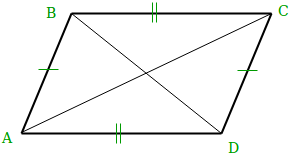# Find all possible coordinates of parallelogram

Find the all the possible coordinate from the given three coordinates to make a parallelogram of a non-zero area.

Let’s call A,B,C are the three given points. We can have only the three possible situations:

```(1) AB and AC are sides, and BC a diagonal
(2) AB and BC are sides, and AC a diagonal
(3) BC and AC are sides, and AB a diagonal ```

Hence, we can say that only 3 coordinates are possible from which we can generate a parallelogram if three coordinates are given.

To prove that all the three points are different let’s suppose it’s wrong. Without losing of generality suppose that the points got in cases AD and BC are equal.
Consider the system of two equations for the equality of these points:

```Bx + Cx - Ax = Ax + Cx - Bx
By + Cy - Ay = Ay + Cy - By

It can be simplified as-

Ax = Bx
Ay = By```

And we got a contradiction, as all the points A, B, C are distinct.

Examples:

```Input  : A = (0 0)
B = (1 0)
C = (0 1)
Output :  1 -1
-1 1
1 1

Input  : A = (-1 -1)
B = (0 1)
C = (1 1)
Output : -2 -1
0 -1
2 3
```

## Recommended: Please try your approach on {IDE} first, before moving on to the solution.Since the opposite sides are equal, AD = BC and AB = CD, we can calculate the co-ordinates of the missing point (D) as:

```AD = BC
(Dx - Ax, Dy - Ay) = (Cx - Bx, Cy - By)
Dx = Ax + Cx - Bx
Dy = Ay + Cy - By```

The cases where the diagonals are AD and BC, CD and AB are processed in the same way.

Reference: https://math.stackexchange.com/questions/1322535/how-many-different-parallelograms-can-be-drawn-if-given-three-co-ordinates-in-3d

Below is the implementation of above approach:

## C++

 `// C++ program to all possible points ` `// of a parallelogram ` `#include ` `using` `namespace` `std; ` `  `  `// main method ` `int` `main() ` `{ ` `   ``int` `ax = 5, ay = 0; ``//coordinates of A ` `   ``int` `bx = 1, by = 1; ``//coordinates of B ` `   ``int` `cx = 2, cy = 5; ``//coordinates of C ` `    ``cout << ax + bx - cx << ``", "` `         ``<< ay + by - cy <

## Java

 `// Java program to all possible  ` `// points of a parallelogram ` `public` `class` `ParallelogramPoints{  ` `     `  `    ``// Driver code ` `    ``public` `static` `void` `main(String[] s) ` `    ``{ ` `        ``int` `ax = ``5``, ay = ``0``; ``//coordinates of A ` `        ``int` `bx = ``1``, by = ``1``; ``//coordinates of B ` `        ``int` `cx = ``2``, cy = ``5``; ``//coordinates of C ` `        ``System.out.println(ax + bx - cx + ``", "`  `                           ``+ (ay + by - cy)); ` `        ``System.out.println(ax + cx - bx + ``", "` `                           ``+ (ay + cy - by)); ` `        ``System.out.println(cx + bx - ax + ``", "` `                           ``+ (cy + by - ax)); ` `    ``} ` `} ` ` `  `// This code is contributed by Prerna Saini `

## Python3

 `# Python3 program to find all possible points ` `# of a parallelogram ` ` `  `ax ``=` `5` `ay ``=` `0` `#coordinates of A ` `bx ``=` `1` `by ``=` `1` `#coordinates of B ` `cx ``=` `2` `cy ``=` `5` `#coordinates of C ` `print``(ax ``+` `bx ``-` `cx, ``", "``, ay ``+` `by ``-` `cy) ` `print``(ax ``+` `cx ``-` `bx, ``", "``, ay ``+` `cy ``-` `by) ` `print``(cx ``+` `bx ``-` `ax, ``", "``, cy ``+` `by ``-` `ax) `

## C#

 `// C# program to all possible  ` `// points of a parallelogram ` `using` `System; ` ` `  `public` `class` `ParallelogramPoints ` `{  ` `     `  `    ``// Driver code ` `    ``public` `static` `void` `Main() ` `    ``{ ` `         `  `        ``//coordinates of A ` `        ``int` `ax = 5, ay = 0;  ` `         `  `        ``//coordinates of B ` `        ``int` `bx = 1, ``by` `= 1;  ` `         `  `        ``//coordinates of C ` `        ``int` `cx = 2, cy = 5;  ` `         `  `        ``Console.WriteLine(ax + bx - cx + ``", "` `                        ``+ (ay + ``by` `- cy)); ` `        ``Console.WriteLine(ax + cx - bx + ``", "` `                        ``+ (ay + cy - ``by``)); ` `        ``Console.WriteLine(cx + bx - ax + ``", "` `                        ``+ (cy + ``by` `- ax)); ` `    ``} ` `} ` ` `  `// This code is contributed by vt_m. `

## PHP

 ` `

Output:

```
4, -4
6, 4
-2, 1
```

Time Complexity: O(1)

Attention reader! Don’t stop learning now. Get hold of all the important DSA concepts with the DSA Self Paced Course at a student-friendly price and become industry ready.

My Personal Notes arrow_drop_upIf you like GeeksforGeeks and would like to contribute, you can also write an article using contribute.geeksforgeeks.org or mail your article to contribute@geeksforgeeks.org. See your article appearing on the GeeksforGeeks main page and help other Geeks.

Please Improve this article if you find anything incorrect by clicking on the "Improve Article" button below.

Improved By : vt_m

Article Tags :
Practice Tags :

Be the First to upvote.

Please write to us at contribute@geeksforgeeks.org to report any issue with the above content.